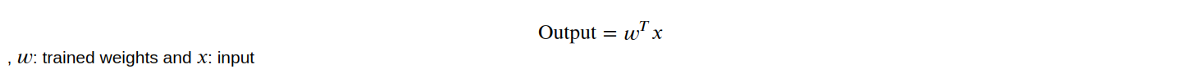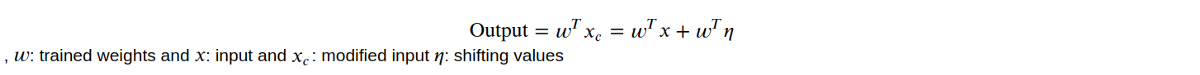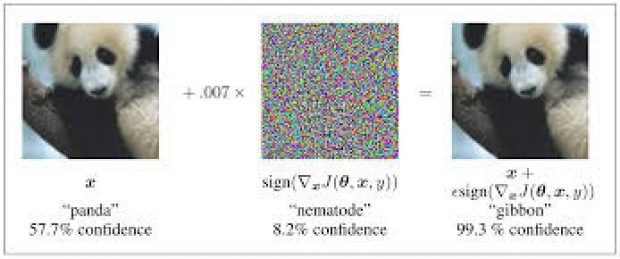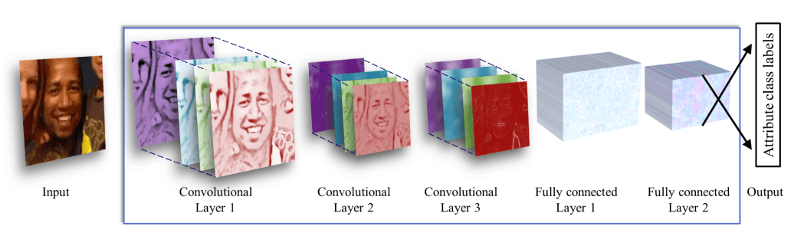Home Technology Convolutional Neural Network How I could hypothetically bypass Account Moderation: Fast Gradient Signed Untargeted on...# How I could hypothetically bypass Account Moderation: Fast Gradient Signed Untargeted on Deep CNN

1751Learn how to use adversarial attacks to not get banned on a dating app just because you’re underage … (we do not endorse the actual application of such method …)I was reading a pretty fun paper recently where they were producing age and gender classifier using a Deep Convolution Neural Network crafted by a team at the University of Israel in their project named “Age Gender Estimation”. Then I thought to myself, what if … I managed to make the network classify a picture of an underage kid as an adult picture? Or an originally very much mature profile picture into an underage picture?Here is the general roadmap of my article:

• 1. What the heck is an adversarial attack (for ML)?
• 2. What is a Fast Gradient Signed Method (FGSM)?
• 3. Is the Age and Gender classifier legit …? (spoiler Yes)
• 4. How to transform your picture to fool the classifier?

## 1. What the heck is an adversarial attack

A formal definition to start with is always better, so here you go! (Wikipedia source…)

Adversarial machine learning is a technique employed in the field of machine learning which attempts to fool models through malicious input. This technique can be applied for a variety of reasons, the most common being to attack or cause a malfunction in standard machine learning models.

Let’s precise the definition to get some more intuition on adversarial attacks in Machine Learning. In our situation, “malicious inputs” refer to images that are images of the actual label but created to “fool” meaning to induce the classifier to think otherwise. So we shift through inputs the correct label to an incorrect label.(TRANSLATION) This is not a smoking pipe

Unexpectedly after some research and few papers reading, I noticed that weaknesses of Machine Learning models are pretty huge, and adversarial attacks to some extent are proof of such statements. The trade-off between making it easy to train, using an approximation of linearity of models and linearity itself being a possible exploitable flaw is even more obvious.

## 2. What is a Fast Gradient Signed Method (FSGM)?

Fast Gradient Signed Method (untargeted) is an adversarial method first published at ICLR 2015 by Ian Goodfellow, Jonathon Shlens, and Christian Szegedy.

It consists of exploiting a general flaw that is the precision value of images. Most images are stored in 0 to 255 i.e. with 8 bits, but then when fed into the Neural Network it is transformed into higher precision values (more accurately, it is given more space). So a trick would be to shift these pixels values enough but less than a certain threshold so as not to change the actual image when rendered while causing the most damage to networks.

So to sum up, we are allowed a small shifting which is a vector (since we are modifying all pixels). But then how should we choose that shifting? (at least how should we pick the direction of this shifting?)

Linear models can ultimately be summed up to such expression.Now assume you have :And what you want is to maximize the last term (in terms of any norm), since you want X_c and X to be as different as possible (again the metrics can be any). Then it is intuitive that you have each coordinate of eta to be of the same sign as their respective coordinate in the vector w.

What interests us more though are non-linear models, since we heavily rely on ReLu, sigmoid in our classifier’s architecture.

With the same idea and after linearizing the cost function we discover that we should be assigning eta’s direction the following way.What remains is then to pick a correct enough norm for the vector eta, and this can be found with a trial and error method.## 3. Is the Age and Gender classifier legit …? (spoiler Yes)

Roughly the architecture works as follows:More precisely it has (it has the same structure as the one in the paper except the adding of two intermediate Conv2D but varies in the number of filters and kernel size):

1. 32 filters of size 3× 3 pixels are applied to the input in the first convolutional layer, followed by a rectified linear operator (ReLU), a max-pooling layer taking the maximal value of 2× 2 regions with one-pixel stride and a local response normalization layer.
2. The 32× 112× 112 output of the previous layer is then processed by the second convolutional layer, containing 64 filters of size 3× 3 pixels. Again, this is followed by ReLU, a max-pooling layer, and a local response normalization layer with the same hyperparameters as before.
3. The third layer operates on the 64 × 53 × 53 blobs by applying a set of 128 filters of size 3 × 3 pixels, followed by ReLU and a max-pooling layer.
4. The fourth layer operates on the 128 × 24 × 24 blobs by applying a set of 256 filters of size 3 × 3 pixels, followed by ReLU and a max-pooling layer.
5. The fifth layer operates on the 256 × 10 × 10 blobs by applying a set of 512 filters of size 3 × 3 pixels, followed by ReLU and a max-pooling layer.
6. A first fully connected layer that receives the output of the fifth convolutional layer and contains 256 neurons, followed by a ReLU and a dropout layer.
7. A second fully connected layer that receives the 512- dimensional output of the first fully connected layer and again contains 512 neurons, followed by a ReLU and a dropout layer.
8. A third, fully connected layer that maps to the final classes for age or gender.

Dataset (called Adience benchmark) for its training came from here again the same entity from the University of Israel. The dataset is composed of 26580 images, with 8 age groups (0–2, 4–6, 8–13, 15–20, 25–32, 38–43, 48–53, 60-) and 2 gender groups.

Note: The source code for the classifier is linked below

Age and Gender Classification Using Convolutional Neural Networks
Published in IEEE Workshop on Analysis and Modeling of Faces and Gestures (AMFG), at the IEEE Conf. on Computer Vision…talhassner.github.io

You can also find more about the project through their arxiv paper.

Since the end goal is to fool a prediction of underage to adult, we grouped the confidence and labels together to form two main classes (adult, not adult). Sample of the classifier output (Figure 1):

Here are some accuracy tests that are at least in our case outperforming the accuracies of from the paper’s architecture of around 45%. (Figure 2)

Note that this accuracy is computed on 8 different classes that are very much short in terms of interval. When aggregated to only 2 classes (adult/not adult) the accuracy increases very much.

## 4. How to transform your picture to fool the classifier?

First of all we load the data and prepare the encoding:

We also prepare the model and load the trained classifier (we could have simply used a json instead of recreating a sequential but here we wanted to show the architecture again)

Now that we have our classifier, we would have to generate an adversarial image to test its confidence. In order to do that, first, we compute the loss between the predicted label of the classifier and the ground truth label, then compute the gradient as a function of the input of this loss. This gives us the direction to go to shift the image. (Figure 3)

What remains is then to add some sort of layer on the image (add pixel by pixel the two images). This layer we create it by multiplying by an epsilon the values. (here we do the same norm shifting for all pixels, but obviously you can also think about some sort of line-search or genetic algorithm to find epsilons that fit each pixel)